Next: Edit Operations Up: Change Detection in Previous: Related Work

# Overview and Preliminaries

In this section, we formulate the change detection problem and split it into two subproblems that are discussed in later sections. We first introduce these problems informally using an example, and then present the formal definitions and terms used in the rest of the paper.

Hierarchically structured information can be represented as ordered trees---trees in which the children of each node have a designated order. We address our problem of detecting and representing changes in the context of such trees. (Hereafter, when we use the term ``tree'' we mean an ordered tree.) We consider trees in which each node has a label and a value.We also assume that each tree node has a unique identifier; identifiers may be generated by our algorithms when they are not provided in the data itself. Note that the nodes that represent the same real-world entity in different versions may not have the same identifier. We refer to the node with identifier x as ``node x'' for conciseness.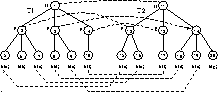Figure 1: Running example

As a running example, consider trees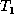and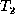shown in Figure 1, and ignore the dashed lines for the moment. The number inside each node is the node's identifier and the letter beside each node is its label. All of the interior nodes have null values, not shown. Leaf nodes have the values indicated in parentheses. (These trees could represent two structured documents, where the labels D, P, and S denote Document, Paragraph, and Sentence, respectively. The values of the sentence nodes are the sentences themselves.) We are interested in finding the delta between these two trees. We will assume thatrepresents the ``old'' data andthe ``new'' data, so we want to determine an appropriate transformation from treeto tree.

Our first task in finding such a transformation is to determine nodes in the two trees that correspond to one another. Intuitively, these are nodes that either remain unchanged or have their value updated in the transformation fromto(rather than, say, deleting the old node and inserting a new one). For example, node 5 inhas the same value as node 15 in, so nodes 5 and 15 should probably correspond. Similarly, nodes 4 and 13 have one child node each, and the child nodes have the same value, so nodes 4 and 13 should probably correspond. The notion of a correspondence between nodes that have identical or similar values is formalized as a matching between node identifiers. Matchings are one-to-one. We say that a matching is partial if only some nodes in the two trees participate, while a matching is total if all nodes participate. Hereafter, we use the term ``matching'' to mean a partial matching unless stated otherwise.

Hence, one of our problems is to find an appropriate matching for the trees we are comparing. We call this problem the Good Matching problem. In some application domains the Good Matching problem is easy, such as when data objects contain object identifiers or unique keys. In other domains, such as structured documents, the matching is based on labels and values only, so the Good Matching problem is more difficult. Furthermore, not only do we want to match nodes that are identical (with respect to the labels and values of the nodes and their children), but we also want to match nodes that are ``approximately equal.'' For instance, node 3 in Figure 1 probably should match node 14 even though node 3 is missing one of the children of 14. Details of the Good Matching problem---including what constitutes a ``good'' matching---are addressed in Section 5. A matching for our running example is illustrated by the dashed lines in Figure 1.

We say that two trees are isomorphic if they are identical except for node identifiers. For treesand, once we have found a good (partial) matching M, our next step is to find a sequence of ``change operations'' that transforms treeinto a tree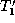that is isomorphic to. Changes may include inserting (leaf) nodes, deleting nodes, updating the values of nodes, and moving nodes along with their subtrees. Intuitively, asis transformed into, the partial matching M is extended into a total matching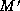between the nodes ofand. The total matchingthen defines the isomorphism between treesand. We call the sequence of change operations an edit script, and we say that the edit script conforms to the original matching M provided that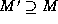. (As will be seen, an edit script conforms to partial matching M as long as the script does not insert or delete nodes participating in M.) Edit scripts are defined in more detail shortly.

We would like our edit script to transform treeas little as possible in order to obtain a tree isomorphic to. To capture minimality of transformations, we introduce the notion of the cost of an edit script, and we look for a script of minimum cost. Thus, our second main problem is the problem of finding such a minimum cost edit script; we refer to this as the Minimum Conforming Edit Script (MCES) problem. The remainder of this section formally defines edit operations and edit scripts. Our algorithm for the MCES problem is presented in Section 4, and Section 5 presents our algorithm for the Good Matching problem. Note that we consider the MCES problem before the Good Matching problem, despite the fact that our method requires finding a matching before generating an edit script. As will be seen, the definition of a good matching relies on certain aspects of edit scripts, so for presentation purposes we consider the details of our edit script algorithms first.Next: Edit Operations Up: Change Detection in Previous: Related Work

Sudarshan S. Chawathe
Wed Jun 19 08:22:34 PDT 1996